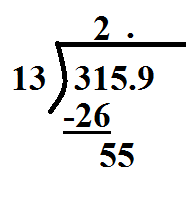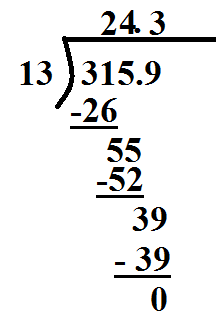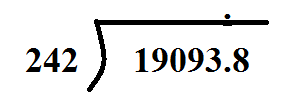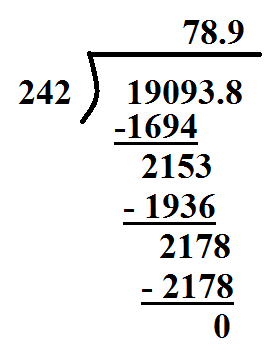# Dividing Decimals

The long division algorithm can be a little tricky to complete. It becomes even more tedious when we include decimals. Let's take a look at how we can make the problem easier.

Type 1: Decimal in the dividend

If you are faced with a decimal inside, you can simply pull the decimal up and divide like it isn't there.

315.9 ÷ 13

Step 1: Pull up the decimal. Be sure to keep it in the same position as it started.Step 2: Start dividing as usual. In this problem, we would start by determining how many times 13 goes into 31 tens. We should get 2 tens. So we will put a 2 in the tens place.Step 3: Continue the process.Here we can see that the quotient is 24.3

Type 2: There is a decimal in the divisor.

The steps are slightly different if there is a decimal in the divisor.

1909.38 ÷ 24.2

It becomes a challenge to try and carry out the long division process when there is a decimal in the divisor. So we will first move it out. To move it out, we multiply by powers of ten.

24.2 x 10 = 242 Now we don't have a decimal in the divisor. However, we cannot just change the value of one of the numbers. This would also change the quotient. So we will do the same change to the dividend.

1909.38 x 10 = 19093.8

Now we are ready to divide: 19093.8 ÷ 242

Start again by pulling up the decimal.Then divide like you normal.At the end, there is no reason to have to move the decimal back. Because you did the same change to both the divisor and dividend, you have still gotten the correct answer. The answer is 78.9. You are finished.